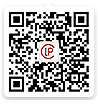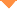首页 > 论文 > 光学学报 > 40卷 > 7期(pp：0727002--1)

Statistical Fluctuation Analysis of Quantum Key Distribution Protocols Based on Heralded Pair Coherent State

• 摘要• 论文信息• 参考文献• 被引情况• PDF全文Abstract

In order to analyze the measurement-device-independent quantum key distribution protocol more comprehensively, the statistical fluctuation analysis of measurement-device-independent quantum key distribution protocols based on heralded pair coherent state is carried out. First, with the increase of the number of transmitted signal pulses in the statistical fluctuation of light source, the relationships of bit error rate and key generation rate with transmission distance are analyzed. The results show that increasing the number of pulses can improve the key generation rate and the maximum transmission distance, and can reduce the bit error rate. Moreover, the measurement-device-independent quantum key distribution protocol based on heralded pair coherent state has better performance than the one based on heralded single photon sources. When the light source is statistical fluctuating, the relationship between the key generation rate and the transmission distance of measurement-device-independent quantum key distribution protocol based on heralded pair coherent state in asymmetric channels is further analyzed, and the simulation results show that this protocol in asymmetric channels has better performance than that in symmetric channels.【1】Mayers D. Unconditional security in quantum cryptography [J]. Journal of the ACM. 2001, 48(3): 351-406.

【2】Gottesman D, Lo H, Lutkenhaus N, et al. Security of quantum key distribution with imperfect devices . [C]//International Symposium on Information Theory, June 27-July 2, 2004, Chicago, IL, USA. New York: IEEE. 2003, 8178599.

【3】Bennett C H, Brassard G. An update on quantum cryptography [M]. //Blakley G R, Chaum D. Advances in Cryptology. Lecture Notes in Computer Science. Berlin: Springer. 1984, 196: 475-480.

【4】Bennett C H, Brassard G, Ekert A K. Quantum cryptography [J]. Scientific American. 1992, 267(4): 50-57.

【5】Wang Q, Wang X B. Efficient implementation of the decoy-state measurement-device-independent quantum key distribution with heralded single-photon sources [J]. Physical Review A. 2013, 88(5): 052332.

【7】Brassard G, Lütkenhaus N, Mor T, et al. Limitations on practical quantum cryptography [J]. Physical Review Letters. 2000, 85(6): 1330-1333.

【8】Zhao Y, Fung C HF, Qi B, et al. Quantum hacking: experimental demonstration of time-shift attack against practical quantum-key-distribution systems [J]. Physical Review A. 2008, 78(4): 042333.

【9】Sun S H, Liang L M. Experimental demonstration of an active phase randomization and monitor module for quantum key distribution [J]. Applied Physics Letters. 2012, 101(7): 071107.

【10】Makarov V, Skaar J. Faked states attack using detector efficiency mismatch on SARG04, phase-time, DPSK, and Ekert protocols [J]. Quantum Information & Computation. 2007, 8(6): 0622-0635.

【11】Lo H K, Curty M, Qi B. Measurement-device-independent quantum key distribution [J]. Physical Review Letters. 2012, 108(13): 130503.

【12】Dong C, Zhao S H, Zhang N, et al. Measurement-device-independent quantum key distribution with odd coherent state [J]. Acta Physica Sinica. 2014, 63(20): 200304.

【13】Sun S H, Gao M, Li C Y, et al. Practical decoy-state measurement-device-independent quantum key distribution [J]. Physical Review A. 2013, 87(5): 052329.

【14】Wang L, Zhao S M, Gong L Y, et al. Free-space measurement-device-independent quantum- key-distribution protocol using decoy states with orbital angular momentum [J]. Chinese Physics B. 2015, 24(12): 120307.

【15】Zhu Z D, Zhao S H, Wang X Y, et al. Phase modulate free measurement device independent quantum key distribution [J]. Journal of Optoelectronics·Laser. 2018, 29(2): 181-186.

【16】Tamaki K, Lo H K. Fung C H Fred, et al. Phase encoding schemes for measurement-device-independent quantum key distribution with basis-dependent flaw [J]. Physical Review A. 2012, 85(4): 042307.

【17】Abruzzo S, Kampermann H, Bruss D. Measurement-device-independent quantum key distribution with quantum memories [J]. Physical Review A. 2013, 89(1): 012301.

【22】Zhang S L, Zou X B, Li C F, et al. A universal coherent source for quantum key distribution [J]. Science Bulletin. 2009, 54(11): 1863-1871.

【23】Wang X, Wang Y, Chen R K, et al. Measurement-device-independent quantum key distribution with heralded pair coherent state [J]. Laser Physics. 2016, 26(6): 065203.

【25】Yu Z W, Zhou Y H, Wang X B. Statistical fluctuation analysis for measurement-device- independent quantum key distribution with three-intensity decoy-state method [J]. Physical Review A. 2015, 91(3): 032318.

【26】Zhou X Y, Zhang C H, Guo G C, et al. The statistical fluctuation analysis for the measurement-device-independent quantum key distribution with heralded single-photon sources [J]. Quantum Information Processing. 2016, 15(6): 2455-2464.

【27】Zhou Y Y, Zhang H Q, Zhou X J, et al. Analysis of the performance of decoy quantum key distribution based on heralded paired coherent state light source [J]. Acta Physica Sinica. 2013, 62(20): 200302.

【28】Dong C, Zhao S H, Shi L. Measurement device-independent quantum key distribution with heralded pair coherent state [J]. Quantum Information Processing. 2016, 15(10): 4253-4263.

【29】Ma X F, Razavi M. Alternative schemes for measurement-device-independent quantum key distribution [J]. Physical Review A. 2012, 86(6): 062319.

He Yefeng,Zhao Yankun,Guo Jiarui,Li Chunyu. Statistical Fluctuation Analysis of Quantum Key Distribution Protocols Based on Heralded Pair Coherent State[J]. Acta Optica Sinica, 2020, 40(7): 0727002